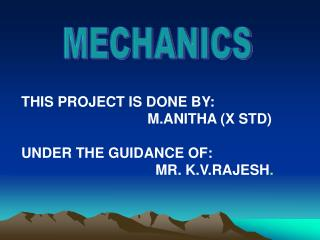# MECHANICS - PowerPoint PPT PresentationDownload PresentationMECHANICS

MECHANICSDownload Presentation## MECHANICS

- - - - - - - - - - - - - - - - - - - - - - - - - - - E N D - - - - - - - - - - - - - - - - - - - - - - - - - - -
##### Presentation Transcript

1. MECHANICS THIS PROJECT IS DONE BY: M.ANITHA (X STD) UNDER THE GUIDANCE OF: MR. K.V.RAJESH.

2. Introduction 1. STABLE EQUILIBRIUM 2. UNSTABLE EQUILIBRIUM 3. NEUTRAL EQULIBRIUM 4. CENTRE OF GRAVITY 5. CONSERVATION OF ENERGY

3. STABLE EQUILIBRIUM : If the forces acting on the body in the displaced position tend to restore it to its original position, the equilibrium is said to be stable. Example: pendulum of a clock, a right circular cone standing on its base, a Pin resting on its head.

4. UNSTABLE EQUILIBRIUM: If the in the displaced position tend to make the body move away from its equilibrium position, the equilibrium is said to be unstable. Example: A pin resting on its point, a cone standing on its vertex.

5. NEUTRAL EQUILIBRIUM: If the body remains in equilibrium even in the displaced position, the equilibrium is said to be neutral. Example: A pin resting on its side, a uniform sphere resting on a horizontal plane, a cone with its slant side on a horizontal plane.

6. CENTRE OF GRAVITY DEFINITION: The center of gravity of a body is the point at which the resultant of the weights of all the particles of the body acts, whatever may be the orientation of the body. The total weight of the body may be supposed to act at its center of gravity. DISTINCTION BETWEEN C.G AND C.M : 1.Now weights of the different particles constituting the Body are proportional to the respective masses. Hence, C.G..,if its exists is the Same as the C.M.. 2.If the body be removed to an infinite distance in space Where the attracting force of the earth is inoperative or if it be imagined to be Taken to the centre of the earth,the force of gravity there will be zero.The body

7. Will lose its weight.Hence,there arises no question of centre of gravity. But the Body will have centre of mass as it will retain its mass which is independent of Gravity and is an inherent property of matter. Thus a body may not have a Centre of gravity but it has a centre of mass. 1.CENTER OF GRAVITY OF A RIGHT CIRCULAR CONE : Distance of the C.G of the cone from the vertex = 3/4 height. 2.CENTER OF GRAVITY OF A HOLLOW RIGHT CIRCULAR CONE : The C.G of the hollow cone is at G such that GL/AL =1/3. 3.CENTER OF GRAVITY OF A SOLID HEMISPHERE : Distance of the G.G of the hemisphere from O is given by =3/8 r.

8. 4.CENTER OF GRAVITY OF A HOLLOW HEMISPHERE : The distance of the C.G of the hollow hemisphere from O is given by = r/2. 5.CENTER OF GRAVITY OF A SOLID TETRAHEDRON : The distance of the center of gravity of the tetrahedron from A = 3/4 height. 6.CENTER OF GRAVITY OF A COMPOUND BODY : W2 G1 G2 G1 G2 = W1 +W2

9. POTENTIAL ENERGY : ‘Potential energy is the energy, possessed by abody by virtue of its position KINETIC ENERGY : Kinetic energy is the energy possessed by a by virtue Of its motion.

10. Conservation Of Energy ‘Energy can neither be created nor be destroyed. It can be transformed from one form into another in an equivalent amount ‘.This is known as the Law Of Conservation Of Energy. When energy is converted from potential energy to kinetic energy, the energy lost in one form reappears in the other. when a body falls, the loss of its potential energy is transformed in kinetic energy till it reaches the ground.